# 14. Models for Nonideal Reactors*

## Topics

1. One Parameter Models
2. Two Parameter Models

 One Parameter Models top

#### A.  Tanks-In-Series

A real reactor will be modeled as a number of equally sized tanks-in-series. Each tank behaves as an ideal CSTR.  The number of tanks necessary, n (our one parameter), is determined from the E(t) curve.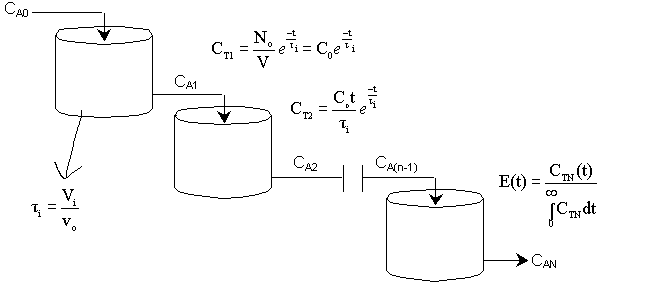For n tanks in series, E(t) is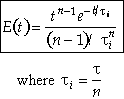It can be shown that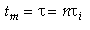In dimensionless form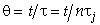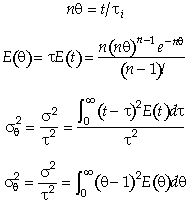Carrying out the integration for the n tanks-in-series E(t)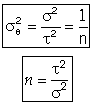For a first order reaction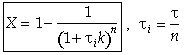For reactions other than first order and for multiple reactions the sequential equations must be solved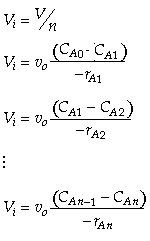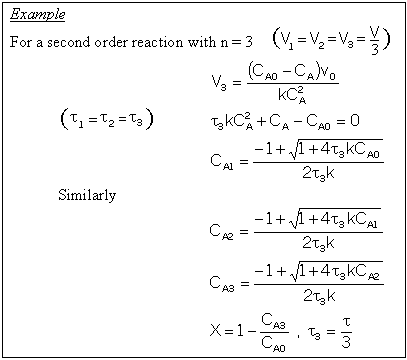#### B.  Dispersion Model

The one parameter to be determined in the dispersion model is the dispersion coefficient, Da. The dispersion model is used most often for non-ideal tubular reactors.  The dispersion coefficient can be found by a pulse tracer experiment.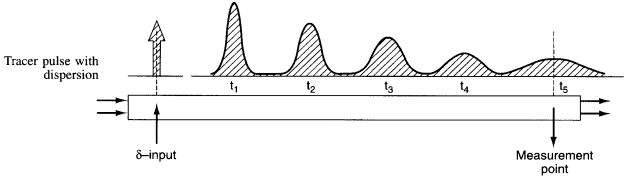After a very, very narrow pulse of tracer is injected, molecular diffusion (and eddy diffusion in turbulent flow) cause to pulse to widen as the tracer molecules diffuse randomly in all directions.  The convective transport equation is: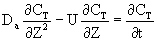Finding the Dispersion Coefficient

1)     For laminar flow Taylor-Aris Dispersion, the molecules diffuse across radial

streamlines as well as axially to disperse the fluid.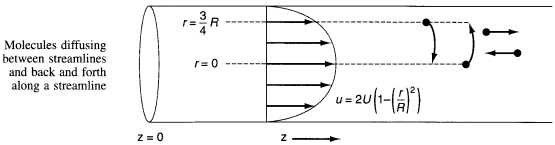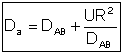2)     To find Da for pipes in turbulent flow see Figure 14-6

3)     To find Da for packed bed reactors see Figure 14-7

Using the RTD to find the Dispersion Coefficient.

Results of the tracer test can be used to determine Per from E(t).  We need to

consider two sets of boundary conditions.

1. Closed-Closed Vessels

2. Open-Open Vessels

For a Closed-Closed Vessel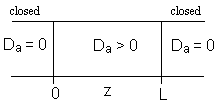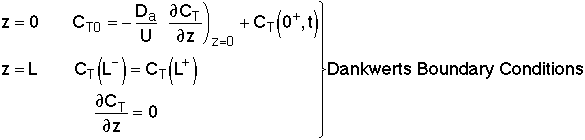We note there is a discontinuity at the entering boundary in the tracer concentration.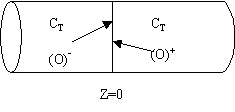Due to the discontinuity at boundary due to forward diffusion,  Ct(O-)=Ct0>Ct(O+)

However, at the end of the reactor,  Ct(L-)=Ct(L+)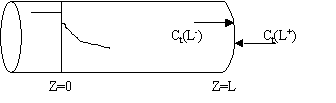Returning to the unsteady tracer balance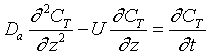(1)

Let λ = z/L,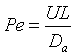andThen in dimensionless form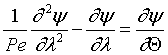For the closed-closed boundary condition the solution to the tracer balance at the exit (λ = 1) , at any time Θ, i.e., Ψ(1,Θ) gives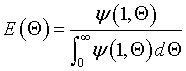where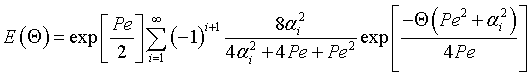(2)

From page 530 of Froment and Bischoff Chemical Reactor Analysis, 2 nd Edition, John Wiley & Sons, 1966.
Eigen Values αi are found from the equation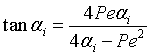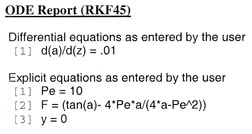For Pe = 10            α1=1.5            α2=2.4            α3=4.8            α4=5.1            α5=7.9            α6=8.1 F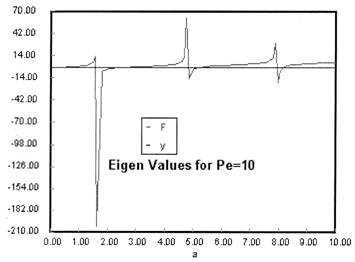0 ≤ λ ≤ 1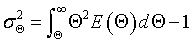Integrating Equation (3) using Equation (2) gives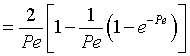(3)

We see from the equation for E(theta) that the exponential term dies out as the values of alpha i become large.

then tm and Per is found from the relationship: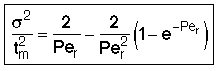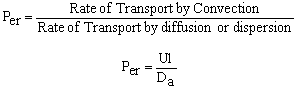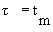Use RTD data to calculate tm,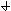2, and then Per (i.e. Da) and then use Da in calculating conversion.

For the solution to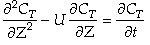with the above boundary conditions we find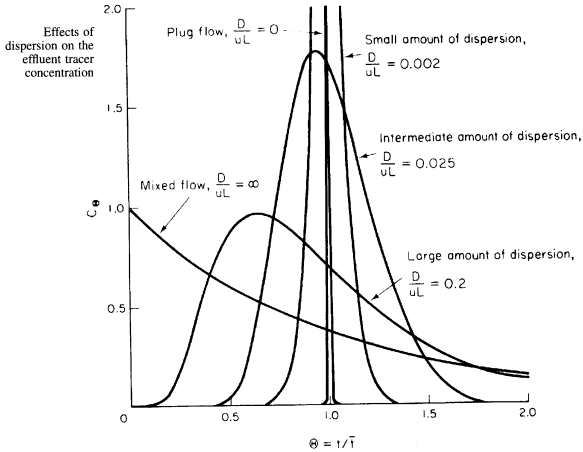For an Open-Open Vessel

Dispersion occurs upstream, downstream and within the reactor.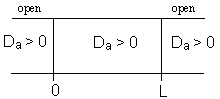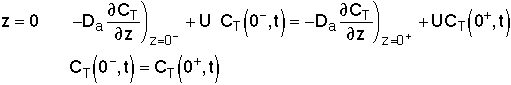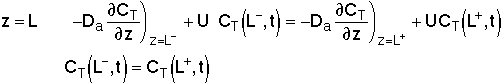Per>100, for long tubes, the solution at the exit isBecause of the dispersion the mean residence time is greater than the space time.  The molecules can flow out of the reactor and then diffuse back in.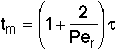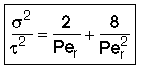Use RTD data to calculate tm,2, and then Per (i.e. Da) and then use Da in calculating conversion. It can be shown that at steady state, the open-open boundary conditions reduce to the Dankwerts Boundary Conditions.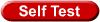Sketch of F curves

#### Dispersion with Reaction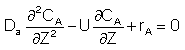For a First Order Reaction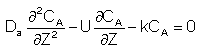Let Ψ = CA/CA0, λ = z/L , Pe = UL/Da , and Da = kτ. The dimensionless balance on the concentration of A in the reaction zone is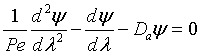Danckwerts Boundary conditions

At λ = 0 then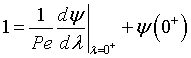At λ = 1, then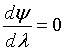the solution is [See John B. Butt, Reaction Kinetics and Reactor Design, 2 nd Edition, page 378, Marcel Dekker, 2000.]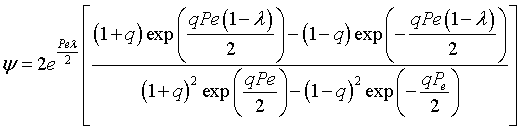where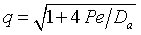The Polymath program used to plot Ψ versus λ is given below

 Polymath Program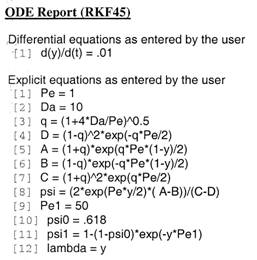Sketches of the dimensionless concentration profiles for different values of Peclet and Damköhler numbers are shown below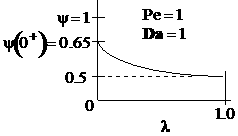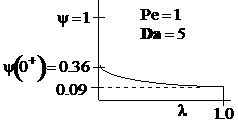Note how Ψ(0+) changes as Pe and Da change.

The exit conversion is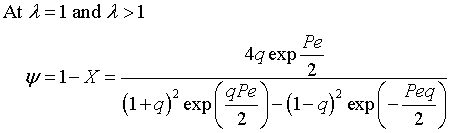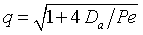The following figures given the Polymath solutions for y versus l for different values of Pe and Da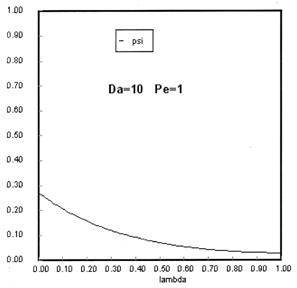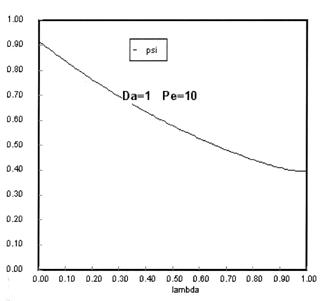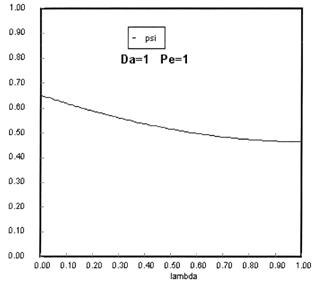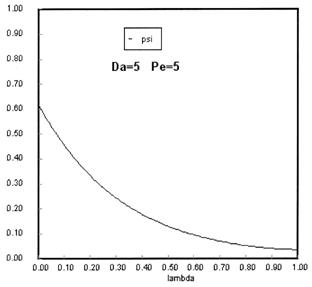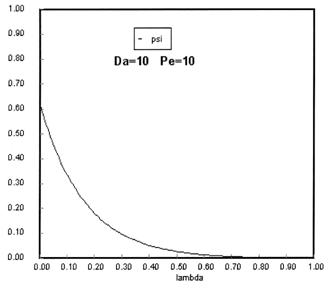Open Open System

Upstream of the reaction zone the balance on A in dimensionless form is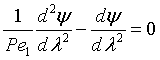For these boundary conditions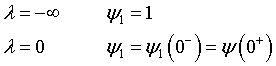the solution is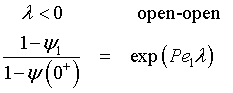Rearranging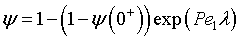A typical profile isThe following profiles were obtained from the Polymath Program given above. Here Ψ1 is the dimensionless concentration of A upstream of the reaction section where Da is greater than zero and Ψ is the dimensionless concentration of A in the reaction section.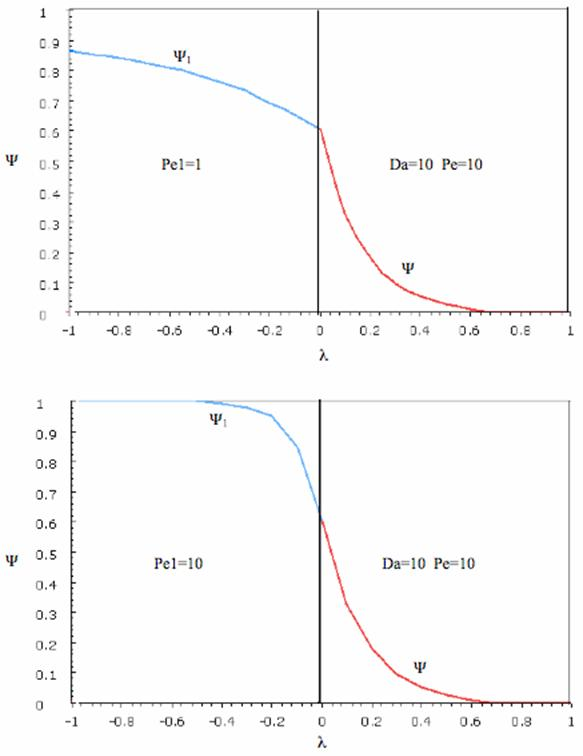Use RTD data to calculate tm,2, and then Per (i.e. Da) and then use Da in calculating conversion.

 Two Parameter Models top

The goal is to model the real reactor with combinations of ideal reactors.

CSTR with Bypass and Dead Volume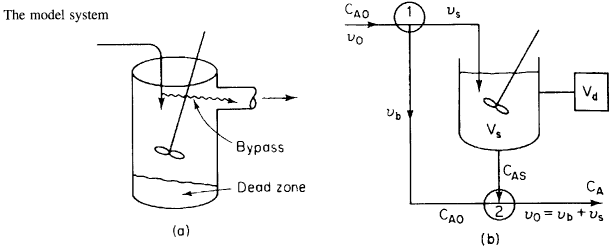Two parameters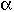and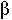, the fraction of volume that is well-mixed (alpha), and the fraction of the stream that is bypassed (beta).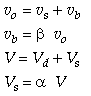#### Reactor Balance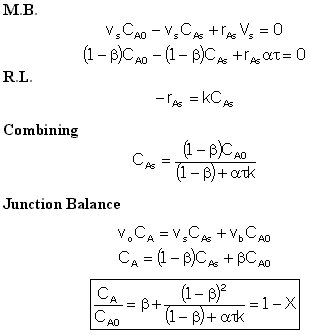We now find the parameters a and b from a tracer experiment. We will choose a step tracer input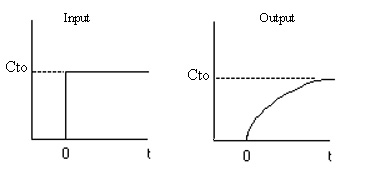The balance equations are: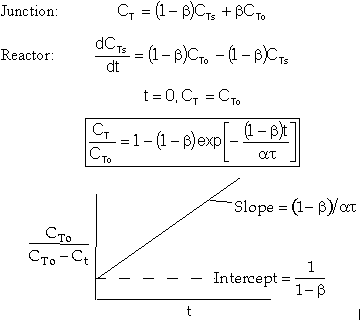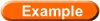Finding a two parameter model

OTHER SCHEME INCLUDED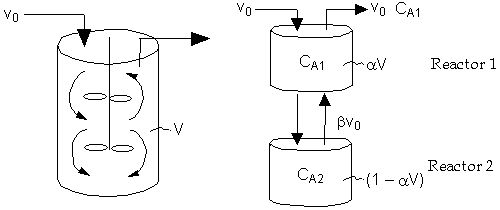Real Reactor                      Model  of Reactor

Two parameters,and:

1. Use the tracer data to findand.

2. Then use the mole balances and the rate law to solve for CA1 and CA2.

 Reactor 1: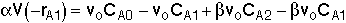(Eqn. A) Rate Law: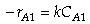for a first order reaction or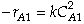for a second order reaction Reactor 2: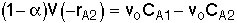(Eqn. B) Rate Law: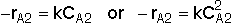Solve Equations (A) and (B) to obtain CA1 as a function of,, k,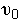, and CA0.

Overall Conversion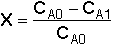Other Scheme For Evaluation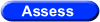Object Assessment of Chapter 14

* All chapter references are for the 4th Edition of the text Elements of Chemical Reaction Engineering .